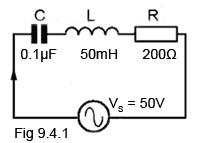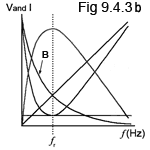# LCR Series Quiz

Try our quiz, based on the information you can find in Module 9. Submit your answers and see how many you get right. If you get answers wrong, just follow the hints on the answers page to find the right answer and learn more about Series LCR circuits as you go.

### 1.With reference to Fig 9.4.1 the resonant frequency of the circuit will be approximately:

### 2.

With reference to Fig 9.4.1, what will be the maximum supply current?

### 3.

With reference to Fig 9.4.1, what will be the approximate voltage across C at resonance?

### 4.

With reference to Fig 9.4.1, what is the Q factor of the circuit?

### 5.

Which of the following statements about a series LCR circuit is true?

### 6.

If the values of L and C in a series LCR circuit are doubled, what will be the effect on the resonant frequency?

### 7.

With reference to Fig 9.4.2, which diagram shows a series LCR circuit at resonance?### 8.

What words are missing from the following statement? The impedance of a series LCR circuit at resonance will be _______ and equal to the circuit _______ .

### 9.With reference to the graph of voltages and current in a series resonant circuit shown in Fig 9.4.3a, What quantity is represented by line A?

### 10.With reference to the graph of voltages and current in a series resonant circuit shown in Fig 9.4.3b, What quantity is represented by line B?